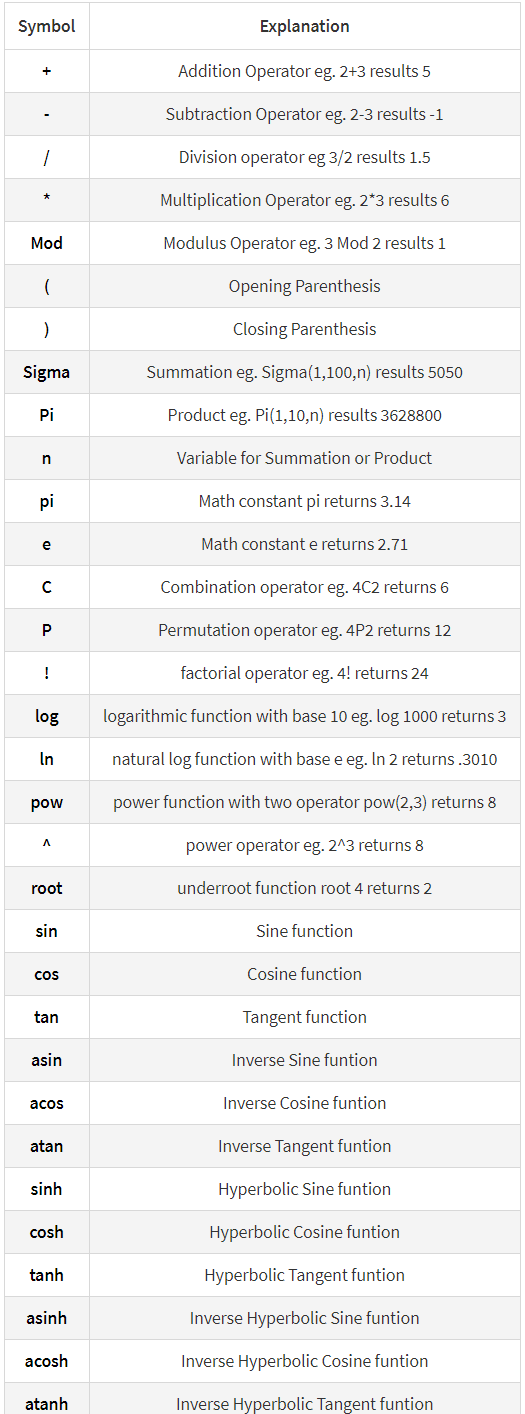## Elementor Form – Calculations

Create form calculators, advanced cost estimators extensive for Elementor Form

With this Add-on for Elementor form you can create advanced calculation forms based on the user input. Make awesome booking and order forms or create complex estimation forms. It is as easy as doing normal mathematics.

#### Conditional calculations

if(A < B,A + 1, A +3 ) + 5 + if(number1 < number2, number-600 + 15, 1) +7

#### Dates calculations

days( date2, date1 )
months( date2, date1 )
year( date2, date1 )

### EQUATIONS / FORMULAS FORMAT FOR CALCULATED FIELDS

With simple mathematical operations:

```sin(cos(tan(90))) * Sigma(1,15,n) + 2^3

fieldname1 / fieldname2

(fieldname1 * ( fieldname2 + fieldname3 ) ) / 5

fieldname1 * ( fieldname2 + fieldname3 )
```

## MAIN FEATURES

• Easy use
• Supports Elementor Free and Elementor Pro Form
• Number and Total formats
• Supports conditional calculations
• Support all mathematical formulas
• Creating forms with automatically calculated fields
• Multi Total field
• Cross browser’s compatible
• Custom format total field
• Finance calculators
• Quote calculators
• Booking cost calculators
• And more…

### Supported symbols### CHANGELOG

```
13/Apr/2022: (version 1.1)